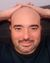# QlikView App Dev

Discussion Board for collaboration related to QlikView App Development.

Announcements
Join us at the Cloud Data and Analytics Tour! REGISTER TODAY
cancel
Showing results for
Search instead for
Did you mean:Contributor III

## Calculate Sum Aggr No distinct

Hi everybody I'm new in the Qlikview Community. I have an issue and I haven’t figured it out yet so maybe you could help me. Here are my datas:

 Product n° Salesman name Qty Qty recalculated Total quantity by product 1 PT 5 5 15 1 PL 5 5 15 1 PM 2 2 15 1 PO 0 3 15 2 PT 9 9 74 2 PL 15 15 74 2 PM 36 36 74 2 PO 14 14 74

I have  this expression for the column Quantity recalculated and it works:

 if(\$(v_Qty_LY)=0,(\$(v_Qty_Total_LY)/\$(v_Nb_Salesman)),\$(v_Qty_LY))

I tried to create an expression to calculate the total quantity by product but it doesn't count the value that replaced the 0.

For example, I don't find 15 but 12. Here is the expression that I created :

 Sum(Aggr(NODISTINCT(\$(v_Qty_recalculated_LY)),Material_Code))

Thanks in advance for your help

Regards

Loreen

1 Solution

Accepted SolutionsMVP

May be try this

`Sum(TOTAL <[Product n°]> Aggr(If(\$(v_Qty_LY) = 0, (\$(v_Qty_Total_LY)/\$(v_Nb_Salesman)),\$(v_Qty_LY)), [Product n°], [Salesman name]))`
3 RepliesCreator II

Hi,

Use a TOTAL qualyfied

`Sum(TOTAL <[Salesman name],[Qty]> \$(v_Qty_recalculated_LY))`

I assume Qty field is a dimension

Ag+

Ag+MVP

May be try this

`Sum(TOTAL <[Product n°]> Aggr(If(\$(v_Qty_LY) = 0, (\$(v_Qty_Total_LY)/\$(v_Nb_Salesman)),\$(v_Qty_LY)), [Product n°], [Salesman name]))`Contributor III
Author

Thank you very much, this solution works.

Have a good day

Regards

Loreen